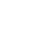# How To Reverse a LinkedListAlex Woods

July 05, 2021

``````class LinkedList:
def __init__(self, value):
self.value = value
self.next = None

# go!
``````

We start out with a linked list like this

``````None   0 -> 1 -> 2 -> 3 -> 4 -> 5
prev   A    B
``````

The variable `head` points at 0.

There are a few constraints when solving this problem

• Your solution should absolutely be `O(n)`
• Use as few variables as possible - management of the pointers is what makes this problem challenging

Walking through each iteration helped me.

``````# after 1 iteration
None <- 0    1 -> 2 -> 3 -> 4 -> 5
prev   A    B
``````

We set `A.next` to `prev`, then iterate forward. The variable `B` really just exists so we can iterate forward once we point `A.next` elsewhere.

``````# after 2 iterations
None <- 0 <- 1    2 -> 3 -> 4 -> 5
prev  A    B

# after 3 iterations
None <- 0 <- 1 <- 2    3 -> 4 -> 5
prev  A    B

# after 4 iterations
None <- 0 <- 1 <- 2 <- 3    4 -> 5
prev  A    B

# after 5 iterations
None <- 0 <- 1 <- 2 <- 3 <- 4    5
prev  A    B

# after 6 iterations
None <- 0 <- 1 <- 2 <- 3 <- 4 <- 5    None None
prev  A    B
``````

Note that `prev` now points at the new `head`.

Here's the final solution.

``````class LinkedList:
def __init__(self, value):
self.value = value
self.next = None

prev = None
b = a.next

while a is not None:
a.next = prev

prev = a
a = b
b = b.next if b is not None else None

return prev
``````

You can get rid of the `if b is not None else None` section if you rearrange the order in which you set everything (you set `b = a.next` before you do `a.next = prev`, but honestly I find that harder to reason about.

This solution has `O(n)` time complexity and `O(1)` space complexity.

Want to know when I write a new article?

Get new posts in your inbox